## 5.10Pressure-velocity coupling

The previous section combined equations for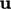and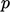, governing momentum and mass conservation, in a sequential solution.

The algorithms used to couple these equations, in a manner which is convergent, uses the following notation to describe terms in the momentum equation, e.g. Eq. (2.67 ), excluding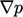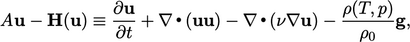(5.16)
where:
•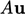is a linear term in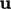;
•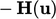is a function ofand other sources.

### Momentum corrector

A momentum corrector equation is formed by expressing the momentum equation, e.g. Eq. (2.67 ) in terms of the notation of Eq. (5.16 ), and rearranging to give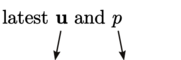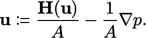(5.17)
The equation provides an update to, based on current values ofandsubstituted on the r.h.s. In other words,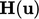and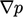are calculated explicitly.

In the algorithms described in the following sections,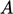is chosen to be the extracted diagonal coeﬃcients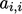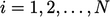of the matrix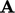corresponding to the momentum terms in Eq. (5.16 ).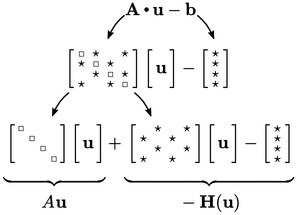The split between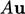andis shown above. As a matrix with diagonal components only,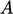has one value per cell so can be represented as a scalar ﬁeld. Settingto be the diagonal coeﬃcients is a natural choice for implicit treatment of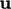within the coupling algorithm.

### Flux corrector

A ﬂux corrector equation follows from Eq. (5.17) by interpolatingto cell faces and evaluating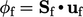according to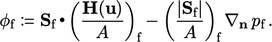(5.18)

### Pressure equation

A pressure equation is then created by substituting ﬂuxes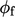from Eq. (5.18 ) into the mass conservation Eq. (2.46 ) in discrete form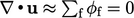. The resulting expression is equivalent to a discretised pressure equation, with coeﬃcients containingand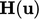,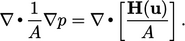(5.19)
Notes on CFD: General Principles - 5.10 Pressure-velocity coupling